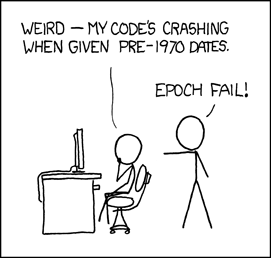# Manual Pages  — CIMAG

### NAME

cimag, conj, cproj, creal – functions to manipulate complex numbers

### LIBRARY

Math Library (libm, -lm)

### SYNOPSIS

`#include <complex.h>`

double
cimag(double complex z);

float
cimagf(float complex z);

long double
cimagl(long double complex z);

double complex
conj(double complex z);

float complex
conjf(float complex z);

long double complex
conjl(long double complex z);

double complex
cproj(double complex z);

float complex
cprojf(float complex z);

long double complex
cprojl(long double complex z);

double
creal(double complex z);

float
crealf(float complex z);

long double
creall(long double complex z);

### DESCRIPTION

Let a, +, b, *, Em, idenote the complex number z.

The creal() functions return the real part a, and the cimag() functions return the imaginary part b.

The conj() functions return the complex conjugate a, -, b, *, Em, i.

The cproj() functions return the projection onto the Riemann sphere. If z contains an infinite component, then the result is , ±, 0, Ns, *, Ns, Em, i, where the (zero) imaginary part of the result has the same sign as b. Otherwise, the result is z.

These functions do not signal any floating point exceptions.

### STANDARDS

The cimag(), conj(), cproj(), and creal() functions conform to ISO/IEC 9899:1999 ("ISO C99").

### HISTORY

The cimag(), conj() and creal() functions first appeared in FreeBSD 5.3 . The cproj() functions appeared in FreeBSD 8.0 .

 CIMAG (3) August 7, 2008